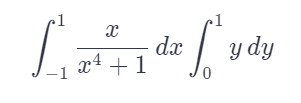# Use Symmetry to Evaluate the Double Integral

We thoroughly check each answer to a question to provide you with the most correct answers. Found a mistake? Let us know about it through the REPORT button at the bottom of the page.

Use symmetry to evaluate the double integral. ∫∫R xy/1+x^4dA, R={(x,y) | -1 ≤x≤1, 0≤y≤1}

## Explanation

First, notice that you can split the integral into the two single variable integrals.The integral involving x may seem troublesome to integrate, but notice that this is an odd function over the symmetric domain with respect to 0. Hence the integral is equal to zero. Since the double integral can be written as a product of two integrals, the total integral is zero.